Courses

# Test: Semiconductor Electronics 4 - From Past 28 Years Questions

## 25 Questions MCQ Test Physics 28 Years Past year papers for NEET/AIPMT Class 12 | Test: Semiconductor Electronics 4 - From Past 28 Years Questions

Description
This mock test of Test: Semiconductor Electronics 4 - From Past 28 Years Questions for Class 12 helps you for every Class 12 entrance exam. This contains 25 Multiple Choice Questions for Class 12 Test: Semiconductor Electronics 4 - From Past 28 Years Questions (mcq) to study with solutions a complete question bank. The solved questions answers in this Test: Semiconductor Electronics 4 - From Past 28 Years Questions quiz give you a good mix of easy questions and tough questions. Class 12 students definitely take this Test: Semiconductor Electronics 4 - From Past 28 Years Questions exercise for a better result in the exam. You can find other Test: Semiconductor Electronics 4 - From Past 28 Years Questions extra questions, long questions & short questions for Class 12 on EduRev as well by searching above.
QUESTION: 1

### p-n junction is said to be forward biased, when      

Solution:

For forward biasing of p-n junction, the
positive terminal of external battery is to be
connected to p-semiconductor and negative
terminal of battery to the n-semiconductor.

QUESTION: 2

### At absolute zero, Si acts as 

Solution:

Semiconductors are insulators at room
temperature

QUESTION: 3

### When n-type semiconductor is heated 

Solution:

Due to heating, when a free electron is
produced then simultaneously a hole is also
produced.

QUESTION: 4

Radiowaves of constant amplitude can begenerated with 

Solution:

Radiowaves of constant amplitude can be
produced by using oscillator with proper
feedback.

QUESTION: 5

In a common base amplifier the phase differencebetween the input signal voltage and the outputvoltage is 

Solution:

The phase difference between output
voltage and input signal voltage in common
base transistor or circuit is zero.

QUESTION: 6

When a triode is used as an amplifier the phasedifference between the input signal voltage andthe output is 

Solution:

Voltage gain of an amplifier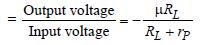voltage differs in phase from the input
voltage by 180°(or π). This holds for a pure

QUESTION: 7

The depletion layer in the p-n junction region iscaused by 

Solution:

The depletion layer in the p-n junction region
is caused by diffusion of charge carriers.

QUESTION: 8

The following truth table corresponds to the logic gate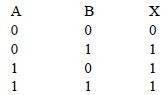Solution:

This truth table is of identity, X = A + B, hence
OR gate.

QUESTION: 9

To use a transistor as an amplifier 

Solution:

To use a transistor as an amplifier the emitter
base junction is forward biased while the
collector base junction is reverse biased

QUESTION: 10

Which one of the following is the weakest kindof bonding in solids 

Solution:

Vander Waal’s bonding is the weakest
bonding in solids

QUESTION: 11

For amplification by a triode, the signal to beamplified is given to 

Solution:

The amplifying action of a triode is based on
the fact that a small change in grid voltage
causes a large change in plate current. The
AC input signal which is to be amplified is
superimposed on the grid potential.

QUESTION: 12

For an electronic valve, the plate current I andplate voltage V in the space charge limited regionare related as 

Solution:

According to Child’s Law,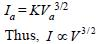QUESTION: 13

A piece of copper and other of germanium arecooled from the room temperature to 80 K, then    

Solution:

Copper is a conductor, so, its resistance
decreases on decreasing temperature as
thermal agitation decreases whereas
germanium is semiconductor, therefore, on
decreasing temperature resistance increases.

QUESTION: 14

Diamond is very hard because 

Solution:

Diamond is very hard due to large cohesive
energy.

QUESTION: 15

The part of the transistor which is heavily dopedto produce large number of majority carriers is 

Solution:

The function of emitter is to supply the
majority carriers. So, it is heavily doped

QUESTION: 16

An oscillator is nothing but an amplifer with      

Solution:

A positive feed back from output to input in
an amplifier provides oscillations of constant
amplitude.

QUESTION: 17

When a p-n junction diode is reverse biased theflow of current across the junction is mainly dueto 

Solution:

When p-n junction is reverse biased, the flow
of current is due to drifting of minority charge
carriers across the junction.

QUESTION: 18

In Fig., the input is across the terminals A and C and the output is across B and D. Then the output is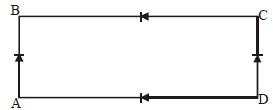Solution:

It is the circuit of full wave rectifier.

QUESTION: 19

Which of the following gates corresponds tothe truth table given below?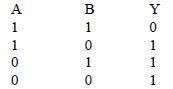Solution:

This truth table is of the identity,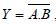hence it is NAND gate.
Here, the output is high even if all inputs are low or one input is low.

QUESTION: 20

In the diagram, the input is across the terminals A and C and the output is across B and D. Then the output is [1994, 02]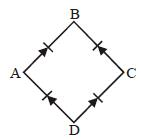Solution:

The given circuit is a circuit of full wave
rectifier.

QUESTION: 21

Which of the following, when added as animpurity, into the silicon, produces n-type semiconductor?   

Solution:

Phosphorous (P) is pentavalent and silicon
is tetravalent. Therefore, when silicon is
doped with pentavalent impurity, it forms a
n-type semiconductor.

QUESTION: 22

The current gain for a transistor working ascommon-base amplifier is 0.96. If the emittercurrent is 7.2 mA, then the base current is  

Solution:

Current gain (α) = 0.96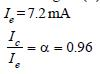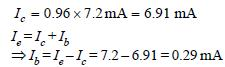QUESTION: 23

When an n–p–n transistor is used as an amplifierthen   

Solution:

In an n-p-n transistor, the charge carriers,
are free electrons in the transistor as well as
in external circuit; these electrons flow from
emitter to collector

QUESTION: 24

When arsenic is added as an impurity to silicon,the resulting material is 

Solution:

Arsenic contains 5 electrons in its outermost
shell. When Arsenic is mixed with silicon
there is one electron extra in silicon crystal.
Hence, such type of semi conductor is ntype
semi conductor

QUESTION: 25

To obtain a p-type germanium semiconductor,it must be doped with   

Solution:

p-type germanium semiconductor is formed
when it is doped with a trivalent impurity
atom.